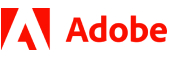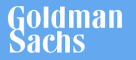New update is available. Click here to update.
Topics

Moderate0/80
Average time to solve is 37m+1 more companies

## Problem statement

You are given a Singly Linked List of integers and an integer 'K'.

Your task is to modify the linked list by inserting a new node after every 'K' node in the linked list with the node value being equal to the sum of the previous 'K' nodes.

Note :
``````If you reach a situation when the number of nodes remaining in the linked list is less than 'K' but greater than zero, just insert a node with the value equal to the sum of the remaining nodes.
``````
Detailed explanation ( Input/output format, Notes, Images )
Constraints:
``````0 <= L <= 5 * 10^5
-10^3 <= data <= 10^3 and data != -1
1 <= K <= 10^6

where 'L' is the number of nodes in the linked list and 'data' is the value of elements present in the given linked list.

Time Limit: 1 sec
``````
Sample Input 1:
``````1 2 3 4 5 6 7 -1
3
``````
Sample Output 1:
``````1 2 3 6 4 5 6 15 7 7 -1
``````
Explanation For Sample Output 1:
``````For the given input, K = 3, so we have inserted a node after the first 3 nodes with a value of 6 as (1 + 2 + 3).

Similarly, we insert a node after the next 3 nodes with a value of 15 as (4 + 5 + 6). Now only 1 node is left (with a value 7) and 0 < 1 < K, so we insert a node with the value 7 at the end of the list.
``````
Sample Input 2:
``````0 6 1 5 -1
2
``````
Sample Output 2:
``````0 6 6 1 5 6 -1
``````Console